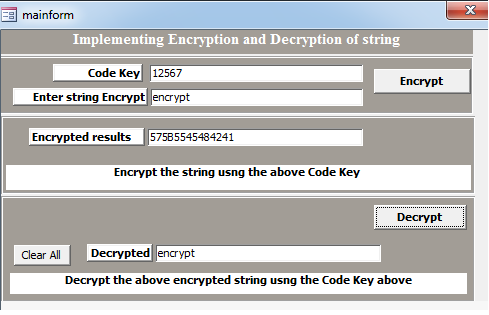# Encrypt and Decrypt data in MS Access using VBA

How to implement Encryption and Decryption using MS Access VBA

This article explains fundamental concept to implement encryption and decryption. Article assumes readers should have good knowledge of VBA coding skills. In order to complete this task we first create a form along with basic controls on it. As shown below in figure Fig-1.1 form includes a buttons ,Labels, input boxes.Fig:-1.1

This article demonstrates XOR cipher technique in order to implement encryption and decryption. In cryptography is a type of additive cipher here in order to encrypt and decrypt a string we need two different values. So first value we are getting from string to be encrypted and second value from code key. Same has been depicted in picture mention below in figure Fig-1.2.Fig:-1.2

After encryption if code value remains intact then only decryption will be successful otherwise it will result into some ambiguous value .Details has been shown in figure Fig-1.3.Fig:-1.3

VBA Code

Now we will associate VBA code with On Click event of button named cmdEncrypt.

Private Sub cmdEncrypt_Click()
Dim codekeydata2 As String
codekeydata2 = 2405
If IsNull(Me.txtCodeKey) Then
Me.txtEncrypted = XOREncryption(codekeydata2, Me.txtInput)
Else
Me.txtEncrypted = XOREncryption(Me.txtCodeKey, Me.txtInput)
End If
End Sub

'Now we will associate VBA code with On Click event of button named cmdDecrypt.
Private Sub cmdDecrypt_Click()
Dim codekeydata As String
Dim txtMessage As String
codekeydata = "2405"
If IsNull(Me.txtCodeKey) Then
Me.txtDecrypted = XORDecryption(codekeydata, Me.txtEncrypted)
Else
Me.txtDecrypted = XORDecryption(Me.txtCodeKey, Me.txtEncrypted)
End If
If Me.txtDecrypted = Me.txtInput Then
txtMessage = "Successfully decrypted"
Else
txtMessage = "Not decrypted successfully"
End If
End Sub
'Now we will associate VBA code with On Click event of button named cmdclr.
Private Sub cmdclr_Click()
Me.txtCodeKey = Nothing
Me.txtInput = Nothing
Me.txtDecrypted = Nothing
Me.txtEncrypted = Nothing
End Sub

'Code contained within module named mdlforencryptionanddecryption
Public Function XORDecryption(CodeKey As String, DataIn As String) As String
Dim arkdata1 As Long
Dim strDataOut As String
Dim intXOrValue1 As Integer
Dim intXOrValue2 As Integer
For arkdata1 = 1 To (Len(DataIn) / 2)
'The first value to be XOr-ed comes from the data to be encrypted
intXOrValue1 = Val("&H" & (Mid\$(DataIn, (2 * arkdata1) - 1, 2)))
'The second value comes from the code key
intXOrValue2 = Asc(Mid\$(CodeKey, ((arkdata1 Mod Len(CodeKey)) + 1), 1))
strDataOut = strDataOut + Chr(intXOrValue1 Xor intXOrValue2)
Next arkdata1
XORDecryption = strDataOut
End Function

Public Function XOREncryption(CodeKey As String, DataIn As String) As String
Dim arkdata1 As Long
Dim strDataOut As String
Dim temp As Integer
Dim tempstring As String
Dim intXOrValue1 As Integer
Dim intXOrValue2 As Integer
For arkdata1 = 1 To Len(DataIn)
'The first value to be XOr-ed comes from the data to be encrypted
intXOrValue1 = Asc(Mid\$(DataIn, arkdata1, 1))
'The second value comes from the code key
intXOrValue2 = Asc(Mid\$(CodeKey, ((arkdata1 Mod Len(CodeKey)) + 1), 1))
temp = (intXOrValue1 Xor intXOrValue2)
tempstring = Hex(temp)
If Len(tempstring) = 1 Then tempstring = "0" & tempstring
strDataOut = strDataOut + tempstring
Next arkdata1
XOREncryption = strDataOut
End Function

DISCLAIMER

It is advised that the information provided in the article should not be used for any kind formal or production programming purposes as content of the article may not be complete or well tested. ERP Makers will not be responsible for any kind of damage (monetary, time, personal or any other type) which may take place because of the usage of the content in the article.